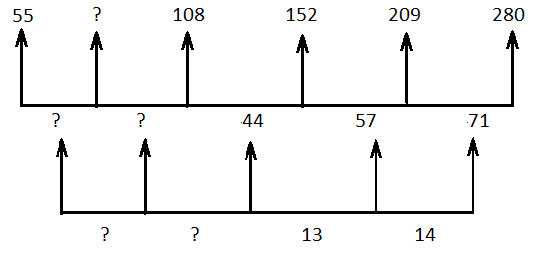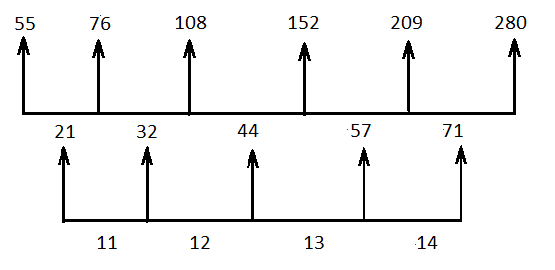Question of The Day23-06-2022

Find the missing number in the series

55, ?, 108, 152, 209, 280.

Correct Answer : d ) 76

Explanation :

Given series is 55, ?, 108, 152 209, 280

As the given series is increasing in a regular manner. So, we will try finding the difference between the numbers to find a pattern.As we can observe in the second step of subtraction, the number is decreasing by 1 from right to left in the second stepTherefore, the missing number of series is 76.

Hence, (d) is the correct answer.0